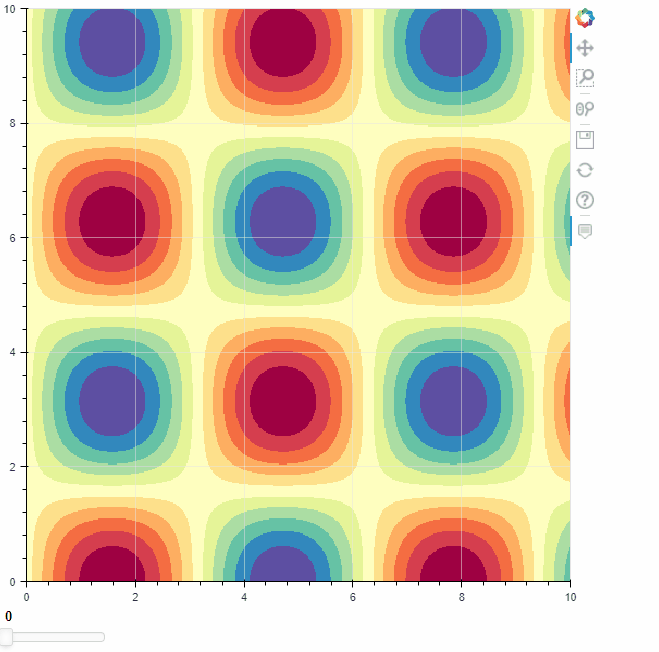# Dynamically updating multiple 2D numpy arrays in Image Glyph

I have written an interactive Bokeh server app that uses two glyphs, the Line Glyph and the Image Glyph, as well as a few sliders.

For the Image Glyph, I store 12 different images in memory and display (3) images on top of each other based on user inputs from various sliders and buttons. Each image is color mapped (red, green, blue) and maintains some amount of transparency to ensure the end user can see the merged result in one Glyph.

My problem is I cannot seem to dynamically update the Image Glyph. All of the online support (tutorials, etc.) recommend leveraging the ColumnDataSource (which I use for the line plots) but ColumnDataSource doesn’t support multiple 2D arrays.

Looking for advice on how to make my Image Glyph dynamic without using ColumnDataSource.

Here’s a basic example showing how to use columndatasource to accomplish this:

``````import numpy as np

from bokeh.plotting import figure, show
from bokeh.layouts import layout
from bokeh.models import Image, ColumnDataSource, Slider, CustomJS

#dummy data taken from https://docs.bokeh.org/en/2.4.0/docs/gallery/image.html
N = 500
x = np.linspace(0, 10, N)
y = np.linspace(0, 10, N)
xx, yy = np.meshgrid(x, y)
#d1 = first image
d1 = np.sin(xx)*np.cos(yy)
#make a second image
d2 = np.sin(xx**2)*np.cos(yy**2)

p = figure(tooltips=[("x", "\$x"), ("y", "\$y"), ("value", "@im")])

#initialize a column datasource and assign first image into it
src = ColumnDataSource(data={'x':,'y':,'dw':,'dh':,'im':[d1]})
#create the image randerer pointing to the field names in src, and the source itself
im_rend = p.image(image='im', x='x', y='y', dw='dw', dh='dh', palette="Spectral11", level="image",source=src)

p.grid.grid_line_width = 0.5

#a widget to put a callback on
sl = Slider(start=0,end=1,value=0,step=1,width=100)

#the key here is to pass a dictionary to the callback all the information you need to UPDATE the columndatasource that's driving the renderer
# imdict is basically this --> if slider value is 0, i want to get d1, if slider value is 1, i want to get d2
cb = CustomJS(args=dict(src=src,imdict={0:d1,1:d2},sl=sl)
,code='''
//assign the im field in the datasource the 2D image associated with the slider value
src.data['im'] = [imdict[sl.value]]
src.change.emit()
''')
sl.js_on_change('value',cb)
lo = layout([p,sl])
show(lo)
``````Now this example only changes the values in the image array, but you could easily expand out imdict to update the other field values in the columndatasource (i.e. the width/height etc.) by just making expanding out imdict to something like:

``````imdict = {0:{'x':,'y':,'dw':,'dh':,'im':[d1]}
,1:{'x':,'y':,'dw':,'dh':,'im':[d2]}}
``````

Then in the callback instead of updating only the ‘im’ field in src, update the columndatasource data entirely:

`src.data = imdict[sl.value]`

Hope this helps!

1 Like

Awesome, thank you!

This topic was automatically closed 90 days after the last reply. New replies are no longer allowed.